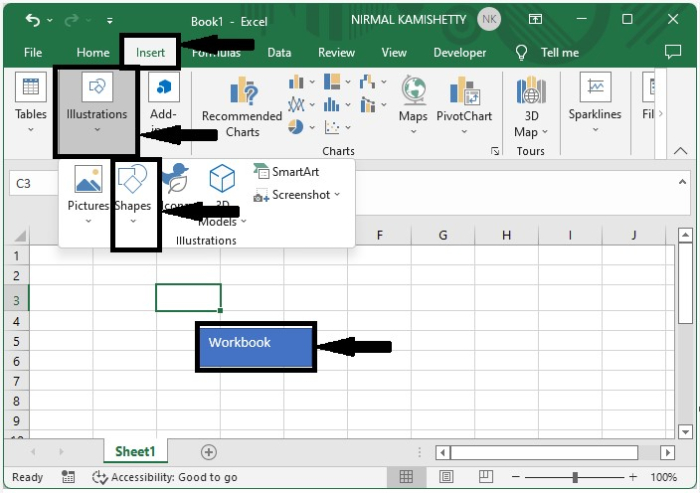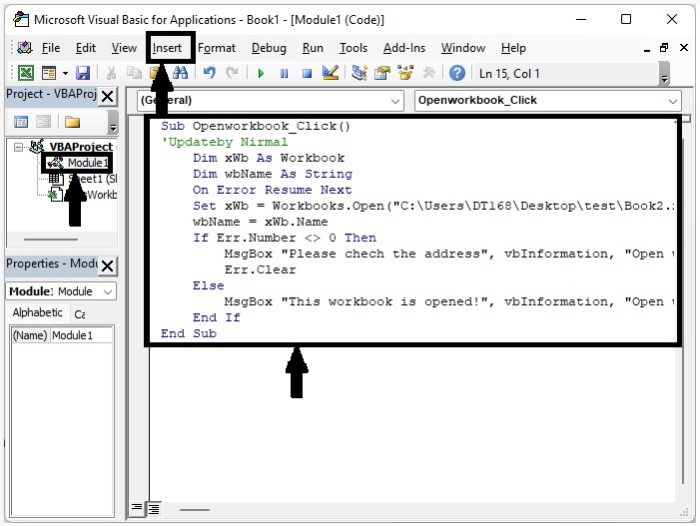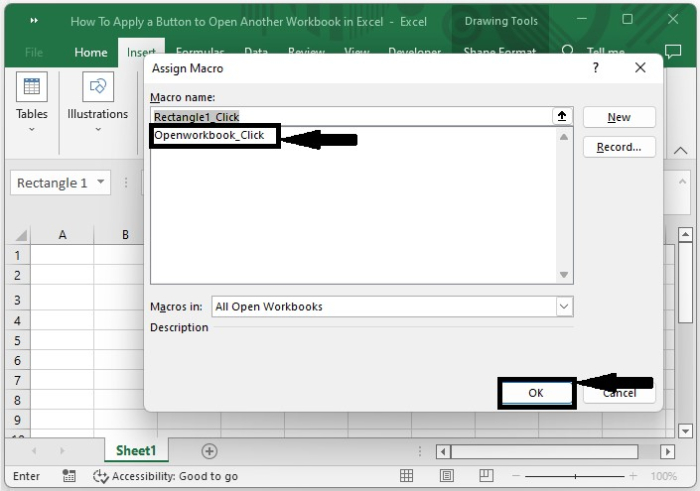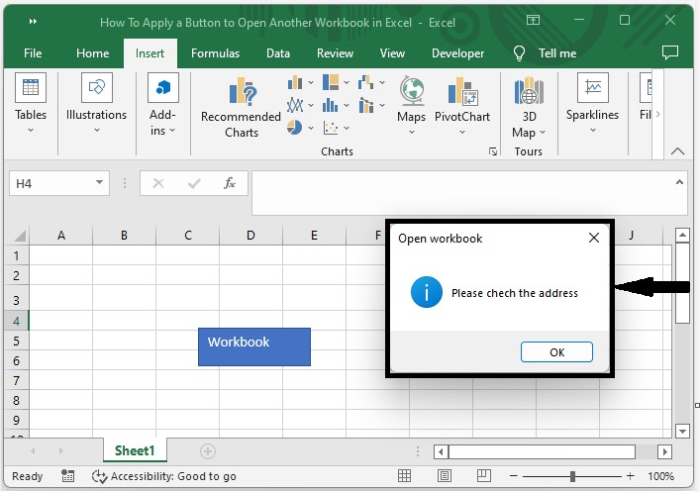# How to Apply a Button to Open Another Workbook in Excel?

Have you ever tried to access the data in two workbooks at once? You could have compared data in two sheets of the same workbook using the VLOOKUP function. We can also connect the two worksheets in Excel. This process can’t be completed using the formulas; we will need to use the VBA code. This tutorial will help you understand how we can apply a button to open another workbook in Excel.

## Applying a Button to Open Another Workbook in Excel

Here we will first insert a shape and then assign a macro to it. Let us see a simple process to see how we can apply a button to open another workbook in Excel.

Step 1

Let us consider a new Excel sheet, then draw a rectangle button on the sheet by clicking "Insert," then "Illustrations," then the shape as shown in the below image.Now right-click on the sheet name and select View Code to open the VBA application. Then in the VBA application, click on insert, select module, and enter the programme in the text window as shown in the below image.

### Program

Sub Openworkbook_Click()
'Updateby Nirmal
Dim xWb As Workbook
Dim wbName As String
On Error Resume Next
Set xWb = Workbooks.Open("C:\Users\DT168\Desktop\test\Book2.xlsx")
wbName = xWb.Name
If Err.Number <> 0 Then
Err.Clear
Else
MsgBox "This workbook is opened!", vbInformation, "Open workbook"
End If
End SubStep 2

Now save the sheet as macro enabled and close the vba application using the command Alt + Q, then right-click on the existing rectangle box in the Excel sheet then select Assign Macro. A pop-up window will then be opened as shown in the below image.Now in the pop-up, select the macro as an open workbook and click on OK to complete our process.

Step 3

If the workbook is not found at the address specified in the code, an error message will be displayed, as shown in the image below.## Conclusion

In this tutorial, we used a simple example to demonstrate how we can apply a button to open another workbook in Excel to highlight a particular set of data.

Updated on: 03-Jan-2023

471 Views